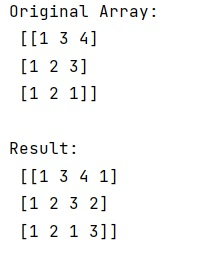# How to add items into a numpy array?

Learn, how to add items into a numpy array in Python?
Submitted by Pranit Sharma, on January 22, 2023

NumPy is an abbreviated form of Numerical Python. It is used for different types of scientific operations in python. Numpy is a vast library in python which is used for almost every kind of scientific or mathematical operation. It is itself an array which is a collection of various methods and functions for processing the arrays.

## Adding items into a numpy array

Suppose we are given a NumPy array that contains some rows and columns of numerical values and we need to add some more values in this array.

We need to add the values in such a way that the values are added to each row of this array. Appending data to an existing array is a natural thing to want to do for anyone with python experience.

We will use the column_stack() method to add a value in each row. This method takes a sequence of 1-D arrays and stacks them as columns to make a single 2-D array. 2-D arrays are stacked as-is, just like with hstack. 1-D arrays are turned into 2-D columns first.

Hence, it will add 1 more column which meant that one new value will be added to each row of this array.

Let us understand with the help of an example,

## Python code to add items into a numpy array

```# Import numpy
import numpy as np

# Creating a numpy array
arr = np.array([[1,3,4],[1,2,3],[1,2,1]])

# Display original array
print("Original Array:\n",arr,"\n")

# Create another array (1D)
b = np.array([1,2,3])

res = np.column_stack((arr,b))

# Display result
print("Result:\n",res,"\n")
```

Output:What's New (MCQs)

Top Interview Coding Problems/Challenges!

IncludeHelp's Blogs

Languages: » C » C++ » C++ STL » Java » Data Structure » C#.Net » Android » Kotlin » SQL
Web Technologies: » PHP » Python » JavaScript » CSS » Ajax » Node.js » Web programming/HTML
Solved programs: » C » C++ » DS » Java » C#
Aptitude que. & ans.: » C » C++ » Java » DBMS
Interview que. & ans.: » C » Embedded C » Java » SEO » HR
CS Subjects: » CS Basics » O.S. » Networks » DBMS » Embedded Systems » Cloud Computing
» Machine learning » CS Organizations » Linux » DOS
More: » Articles » Puzzles » News/Updates